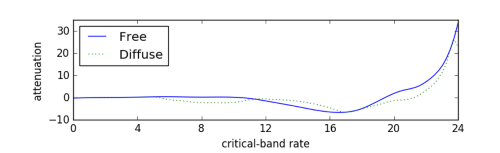# How to Digitize a Graph

### Sometimes life throws you a curve and you need to draw some conclusions. Here's an easy-to-use Python method to help you. Warning: The following content is graphic.

· Web Dev Zone · Tutorial
Save
8.19K Views

suppose you have a graph of a function, but you don’t have an equation for it or the data that produced it. how can you reconstruction the function?

there are a lot of software packages to digitize images. for example, web plot digitizer is one you can use online. once you have digitized the graph at a few points, you can fit a spline to the points to approximately reconstruct the function. then as a sanity check, plot your reconstruction to see if it looks like the original. it helps to have the same aspect ratio so you’re not distracted by something that doesn’t matter, and so that differences that do matter are easier to see.

for example, here is a graph from zwicker and fastl’s book on psychoacoustics. it contains many graphs with no data or formulas. this particular one gives the logarithmic transmission factor between free field and the peripheral hearing system.here’s python code to reconstruct the functions behind these two curves.

``````import matplotlib.pyplot as plt
import numpy as np
from scipy import interpolate

curve_names = ["free", "diffuse"]
plot_styles = { "free" : 'b-', "diffuse" : 'g:'}

data = {}
for name in curve_names:

x = data[:,0]
y = data[:,1]
spline = interpolate.splrep(x, y)
xnew = np.linspace(0, max(x), 100)
ynew = interpolate.splev(xnew, spline, der=0)
plt.plot(xnew, ynew, plot_styles[name])

logical_x_range  = 24    # bark
logical_y_range  = 40    # db
physical_x_range = 7     # inch
physical_y_range = 1.625 # inch

plt.legend(curve_names, loc=2)
plt.xlabel("critical-band rate")
plt.ylabel("attenuation")
plt.xlim((0, logical_x_range))

plt.axes().set_aspect(
(physical_y_range/logical_y_range) /
(physical_x_range/logical_x_range) )
ax = plt.gca()
ax.get_xaxis().set_ticks([0, 4, 8, 12, 16, 20, 24])
ax.get_yaxis().set_ticks([-10, 0, 10, 20, 30])

plt.show()

``````

here’s the reconstructed graph.Topics:
graphs, python, plotting

Published at DZone with permission of John Cook, DZone MVB.

Opinions expressed by DZone contributors are their own.